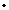PEOPLE Postdoctoral Fellow
International Centre for Theoretical Sciences, Bengaluru
Area of Research:  Mathematical Fluid Dynamics: stability of incompressible fluid models, spectral and eigenvalue problems in hydrodynamics
+91 80 4653 6168
H-108
shibivasudevanictsresin

I study stability problems in incompressible, inviscid fluid models such as the two dimensional Euler equations and regularized variants like the alpha Euler equations using tools from functional analysis, spectral theory and operator theory. I am also interested in other problems arising from fluid dynamics that are amenable to mathematical analysis such as wind driven water waves.